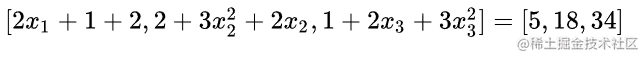# Pytorch中的vector-Jacobian product

an engine for computing vector-Jacobian product

### Jacobian matrix（雅可比矩阵）

Y = G（X） Y是一个向量，X是一个向量，Y对X求导结果就是雅可比矩阵，即 雅可比矩阵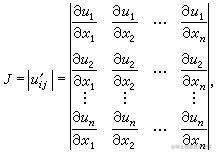### 例子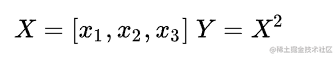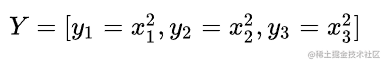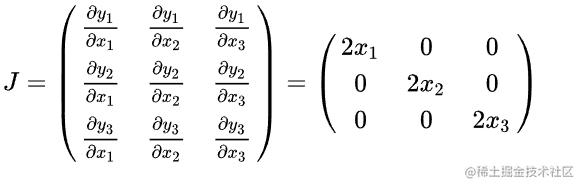### Torch代码验证

``````>>> x1=torch.tensor(1, requires_grad=True, dtype = torch.float)
>>> x2=torch.tensor(2, requires_grad=True, dtype = torch.float)
>>> x3=torch.tensor(3, requires_grad=True, dtype = torch.float)
>>> y=torch.randn(3) # produce a random vector for vector function define
>>> y=x1**2+2*x2+x3 # define each vector function
>>> y=x1+x2**3+x3**2
>>> y=2*x1+x2**2+x3**3
>>> y.backward(torch.ones(3))
tensor(5.)
tensor(18.)
tensor(34.)``````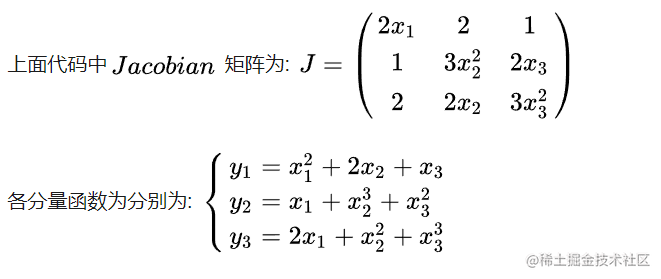J*v =